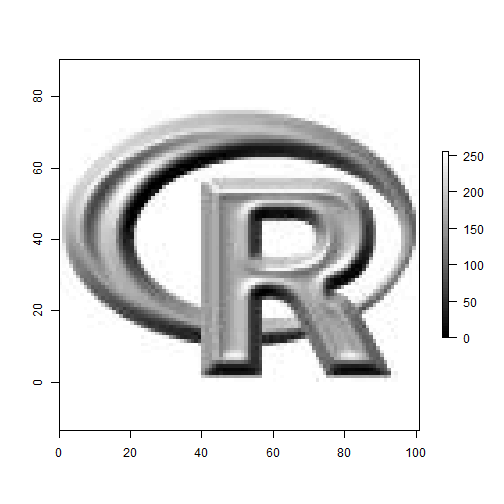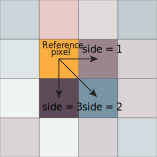Want to share your content on R-bloggers? click here if you have a blog, or here if you don't.

References

In my recent projects, I had the opportunity to work with the professor Raphaël Proulx who introduced me to several metrics commonly used in landscape ecology for quantifying image texture. In order to make my life easier, I decided to implement them as a R package. At this time, the package is still under development and so better documentation/debugging/testing are still needed. Nevertheless, here is a short tutorial on how to use the package.

Step 1: Install the package

The package is currently hosted on Bitbucket and can be installed using devtools.

library(devtools)install_bitbucket("imagemetrics", "persican")library(imagemetrics)

Step 2: Open a raster image

Indeed, the first thing to do is to open the image on which you want to calculate the metrics.

## Open the R logo and average on calculate the average on R,G,B channelsr = brick(system.file("external/rlogo.grd", package = "raster"))r = mean(r)## Plot the raster (optional)plot(r, useRaster = FALSE, col = gray((0:100)/100))Step 3: Extract pixels from the image to calculate occurrence probabilities

It is worth mentioning that the metrics are calculated on a probability matrix that represents the chances of getting a specific pair of pixel values. For each pixel in the image, we have to choose a neighbor that is located either to the right, bottom right or bottom of a reference pixel (see the following image).Before calculating such probabilities, we have to extract the values of both reference and neighbor pixels. To do so, the user can use getImagePixels(r, side). The function takes as parameters a raster image (r) and side, a numeric value specifying the neighbor to use. side = 1 for the right pixel, side = 2 for the lower right pixel, side = 3 the bottom pixel.

## Get bottom pixel (side = 3)v = getImagePixels(r, side = 3)str(v)
## List of 2##  $reference_vector: num [1:7676] 255 255 255 255 255 255 255 255 255 255 ...##$ neighbour_vector: num [1:7676] 255 255 255 255 255 255 255 255 255 255 ...

Step 4: Calculate occurrence probabilities

To calculate the probabilities, simply use calculateHisto(reference_vector, neighbour_vector, nbins, where reference_vector and neighbour_vector are vectors returned by getImagePixels and nbins is a numerical value indicating the bin size used to compute histograms.

From Mellin et al. 2012:

“For $$M$$ classes of values, the number of possible configurations in a $$k-pixel$$ neighborhood is $$M^k$$. To ensure that each possible configuration has a reasonable probability of occurring in an image, it is generally recommended that the ratio of the total number of pixels in the image to $$M^k$$ be greater than 100.”

suggestMaximumBins® can be used to determine the number of maximum bins to use.

suggestMaximumBins(r)
##  8
## Calculate probabilityr.prob = calculateHisto(reference_vector = v$reference_vector, neighbour_vector = v$neighbour_vector,     nbins = 6)

Step 5: Compute the metrics

Metrics are thereafter calculated using the object returned by calculateHisto.

contagion(r.prob)
##  0.3469
contrast(r.prob)
##  0.02727
evenness(r.prob)
##  0.8393
homogeneity(r.prob)
##  0.848
jointEntropy(r.prob)
##  2.341
marginalEntropy(r.prob)
##  1.504
maximumMutualInformation(r.prob)
##  0.3724
meanInformationGain(r.prob)
##  0.4669

## References

Mellin, C., Parrott, L., Andréfouët, S., Bradshaw, C.J.A. a, MacNeil, M.A. & Caley, M.J. (2012) Multi-scale marine biodiversity patterns inferred efficiently from habitat image processing. Ecological Applications, 22, 792803.

Proulx, R. & Parrott, L. (2008) Measures of structural complexity in digital images for monitoring the ecological signature of an old-growth forest ecosystem. Ecological Indicators, 8, 270284.